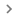# Add & edit a trendline

Before you create a trendline: You can add trendlines to bar, line, column, or scatter charts.

2. Double-click a chart.
3. At the right, click CustomizeSeries.
4. Optional: Next to "Apply to," choose the data series you want to add the trendline to.
5. Click Trendline. If you don’t see this option, trendlines don’t work with your data.
Make changes to a trendline
2. Double-click a chart.
3. At the right, click CustomizeSeries.
4. Optional: Next to "Apply to," choose the data series you want to add the trendline to.
5. Under "Trendline," you can edit:
• Trendline types.
• Line color, opacity, or thickness.
• Labels.
• R squared. This shows how closely the trendline fits the data. The closer to R^2 = 1, the closer the fit. This is only available if you add a legend.
• Polynomial degrees. This is only available for polynomial trendlines.
• Average types. This is only available for moving average trendlines.
• Periods. This is only available for moving average trendlines.
Trendlines & equations to use
• Linear: For data that closely follows a straight line.
• Trendline equation: y = mx+b.
• Exponential: For data that rises and falls proportional to its current value.
• Trendline equation: y = A*e^(Bx).
• Polynomial: For data that varies.
• Trendline equation: ax^n + bx^(n-1) + … + zx^0.
• Logarithmic: For data that rises or falls at a fast rate and then flattens out.
• Trendline equation: y = A*ln(x) + B.
• Power series: For data that rises or falls proportional to its current value at the same rate.
• Trendline equation: y = A*x^b.
• Moving average: Helps smooth out data that is unstable or more variable.